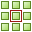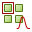# PP Card

This card defines the phase shift of the excitation between one unit cell and the next for periodic boundary conditions. The unit cell for a PBC calculation is specified with the PE card.

On the Home tab, in the Planes / arrays group, click thePeriodic boundary icon. From the drop-down list, click thePeriodic phase (PP) icon.

## Parameters:

Manually specify phase shift
The phase shift is manually specified.
Determine phase shift from plane-wave excitation
When a plane wave is used as excitation the phase difference between the cells cannot be specified, but is determined by the excitation.
Determine phase shift from beam pointing (“squint”) angle:
The phase shift is determined by specifying the theta and phi angle of the “squint” angle.
Phase shift in ${\stackrel{^}{u}}_{1}$ direction (degrees)
Phase shift in the first direction, ${\stackrel{^}{u}}_{1}$ .
Phase shift in ${\stackrel{^}{u}}_{2}$ direction (degrees)
Phase shift in the second direction, ${\stackrel{^}{u}}_{2}$ .

## One dimension:

Theta angle (degrees)
Orientation of the squint angle. The angle, theta, in degrees is the angle between the squint angle and the plane defined by the ${\stackrel{^}{u}}_{1}$ vector.

## Two dimensions:

Theta angle (degrees)
Orientation of the squint angle. The angle, theta, in degrees is the angle between the squint angle and the ${u}_{1}=0$ plane.
Phi angle (degrees)
Orientation of the squint angle. The angle, phi, in degrees is the angle between the squint angle and the plane defined by the ${\stackrel{^}{u}}_{1}$ vector.
Note that multiple PP cards can be used in a model but only one PE card can be used.